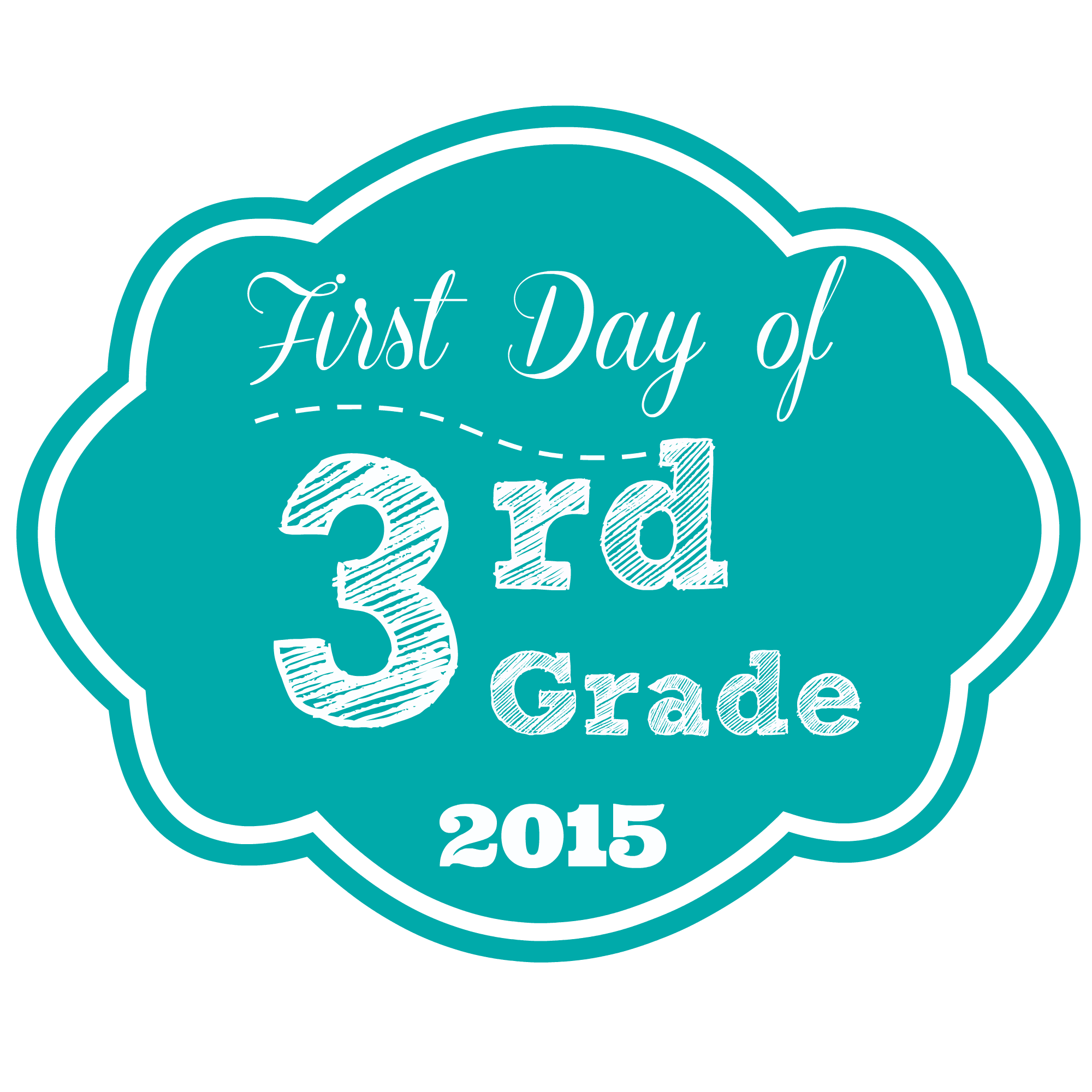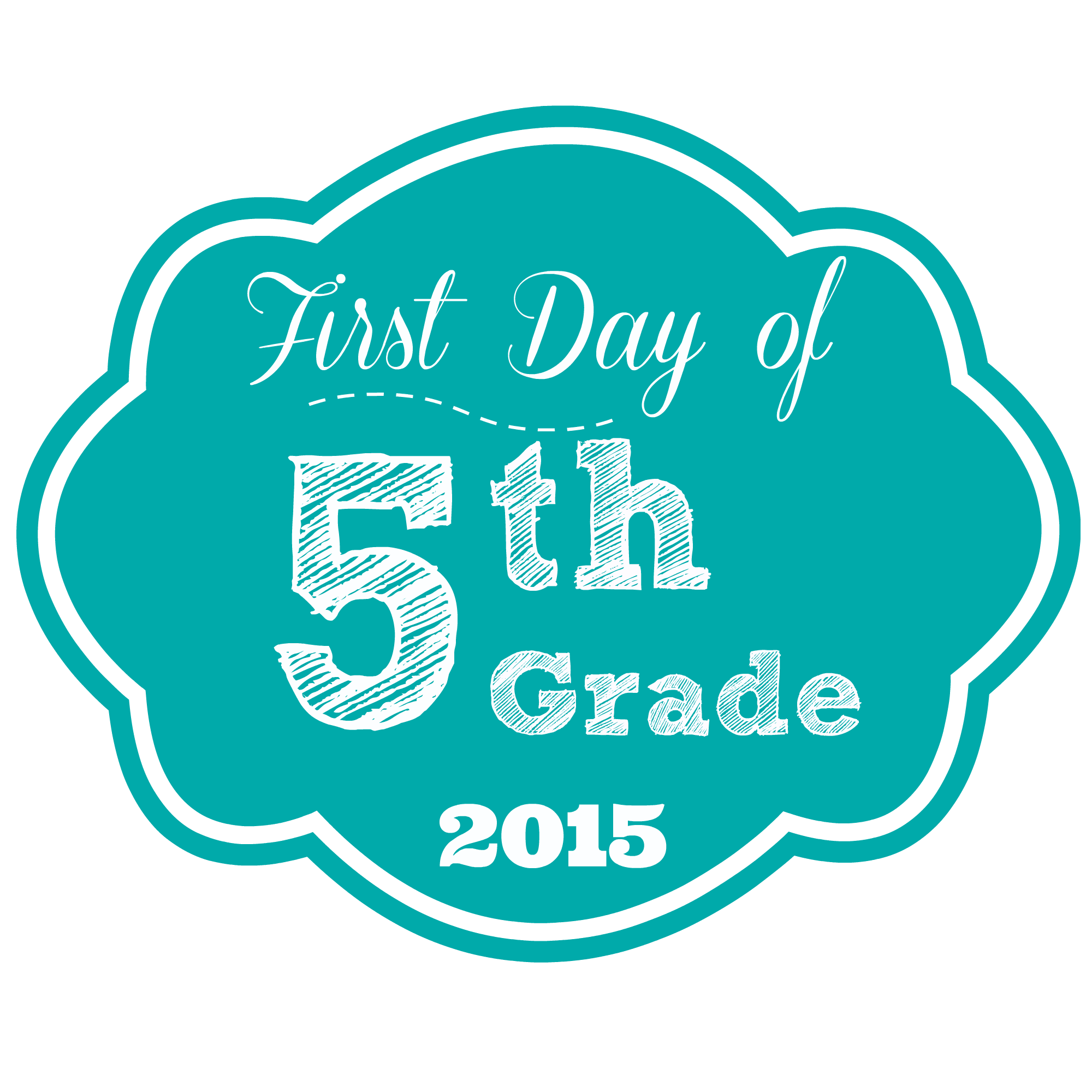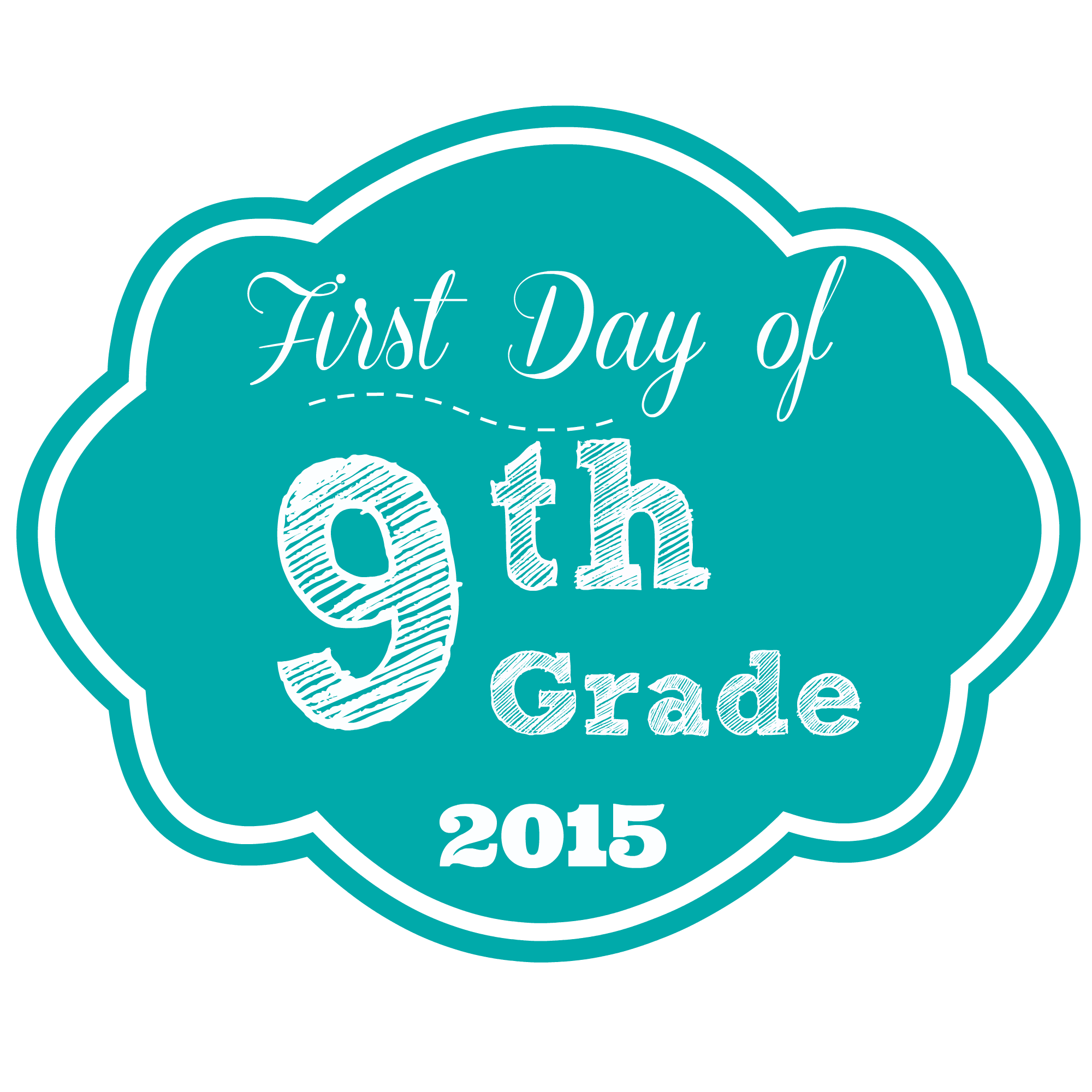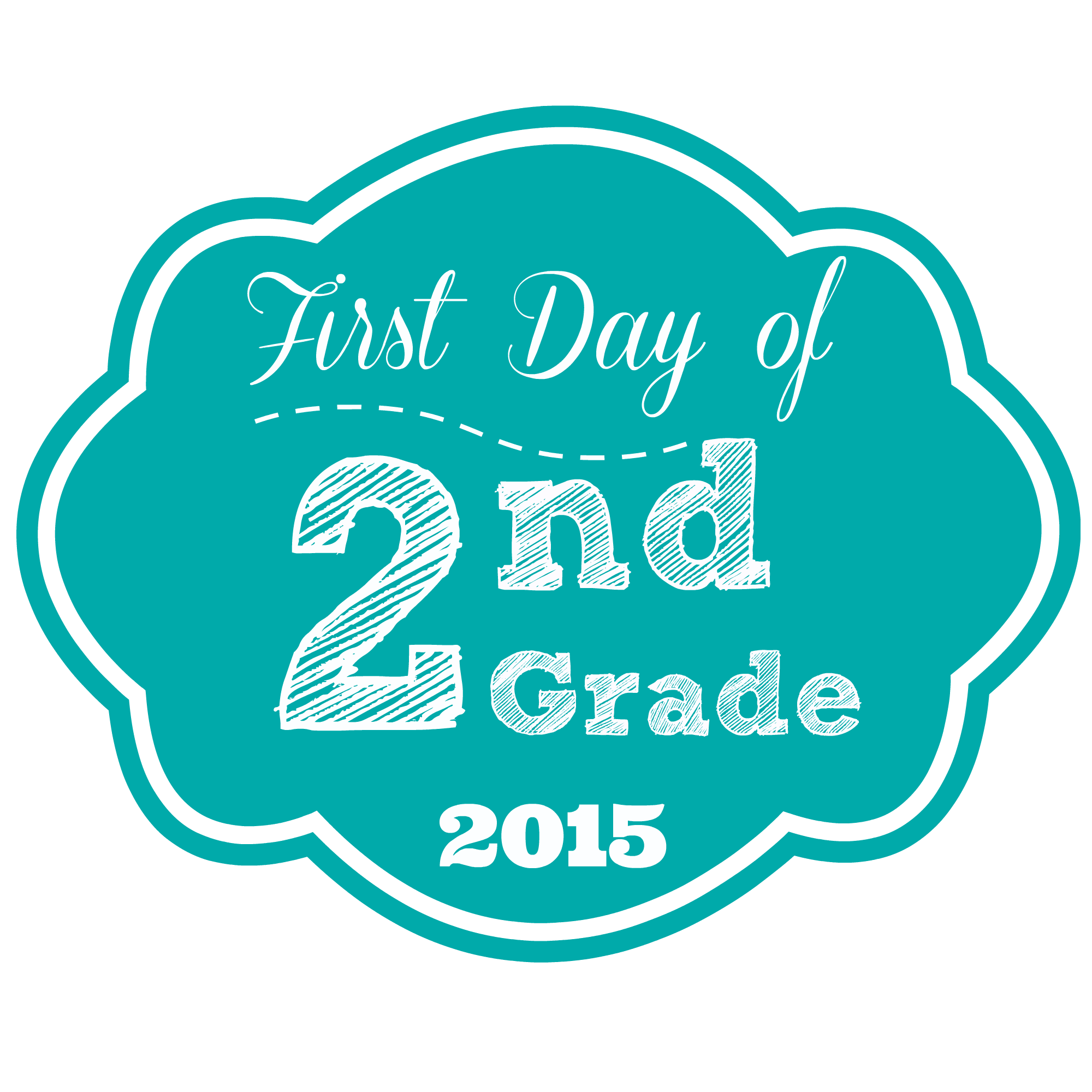Cute School Picture Prop August 5, 2015 08:40

Yay! The kids are going back to school. If you are a parent then you must be excited about it right? LOL Well anyways we have been really busy since we are going to be giving out Free Backpacks at our shoe store for our customers this coming No Tax weekend. Personally I am so looking forward to seeing the children's smiles when they get a backpack. It's very rewarding <3 well="" today="" i="" will="" actually="" share="" with="" you="" not="" an="" outfit="" but="" a="" little="" something="" made="" that="" think="" look="" very="" cute="" in="" your="" children="" s="" first="" day="" of="" school="" pictures="" am="" sure="" many="" take="" child="" on="" their="" especially="" during="" years="" here="" labels="" have="" transparency="" the="" background="" and="" can="" paste="" it="" picture="" photo="" editor="" also="" print="" out="" label="" cut="" glue="" to="" foam="" board="" ice="" cream="" stick="" back="" so="" hold="" as="" prop="" when="" either="" way="" this="" would="" 3="" save="" following="" images="" or="" if="" need="" original="" png="" file="" send="" us="" email="" customerservice="" sarichka="" com="" tell="" which="" one="" want="" be="" more="" than="" happy="" them="" do="" use="" please="" love="" see="" how="" they="" p="">